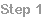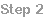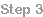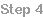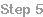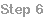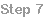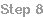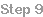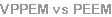Step 8
Our basic explanation is split into several steps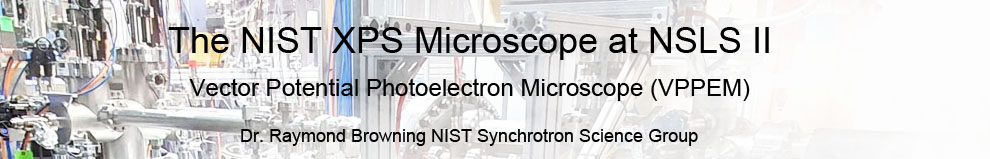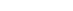The raw VPPEM images need to be processed for analysis and presentation.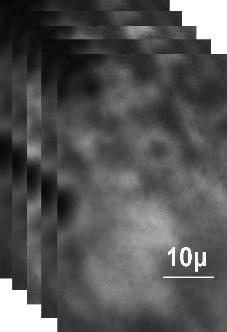Raw image stack with each image using a different
photon energy across the NEXAFS spectrum.
VPPEM produces an image at each step in the NEXAFS spectrum.

If we have several NEXAFS spectra taken from one sample, say from Fe, Cr, NI,
O, and C, then we have multi-hyper-spectral data. We can also change the
sampling energy at each step so each pixel is a two dimensional spectrum.

This gives us a very complex image, and image processing is an integral part
of the experimental technique.

Our approach is heuristic. One fact that we start with, is that although the
multi-hyper-dimensional images are very complex, the samples themselves
have a limited number of unique areas.

The main technique we use is interactive factor analysis.

Interactive factor analysis relies on the operator picking areas of interest in the
raw images, and creating factor images based on the spectra from those
regions.

For example in the image on the right picking one or two bright areas, and one
or two dark areas, and using the spectra from these areas to make a factor
image.
We then use the factor images to create scatter diagrams. From the scatter
diagrams we can see the real complexity of the images.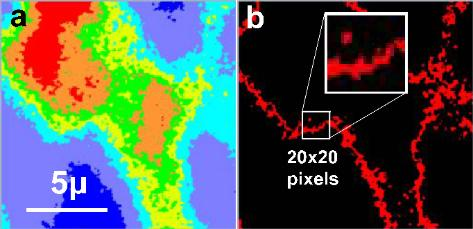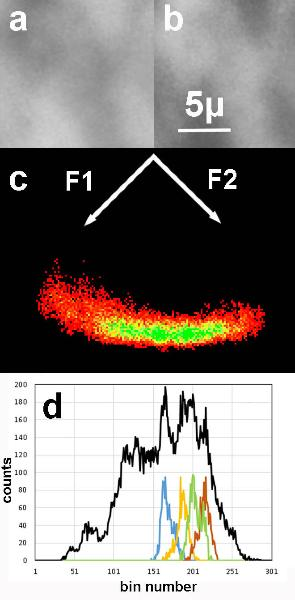The two factor images F1 and  F2 in the left hand panel a and b
give the scatter digram in c. Note this is rotated round 45
degrees.

The distribution along the horizontal axis d shows there are
several distinct features. These can be imaged by windowing
the bin numbers. The windowed image is shown on the right.

Each color has a spectrum associated with it. So that the
chemistry can be identified.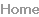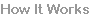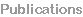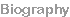How it works